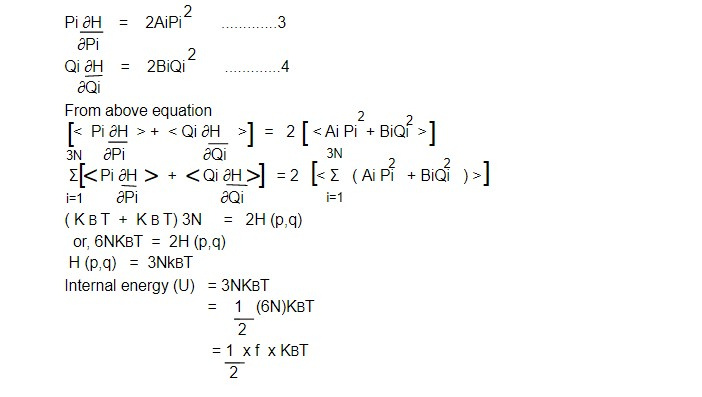# Theorem of equipartition of energy (Boltzman equipartition)

From generalized equipartition theorem

< xi, ∂H/∂xj > = ðij KBT...................1

Many physical system have Hamiltonian which in classical transformation can be expressed aswhere Ai and Bi are constant and Pi and Qi are cannonically conjugate variable for such system.

Now from eqn 2

∂H/∂pi = 2 AiPi and ∂H/∂Qi = 2BiQiwhere f is degree of freedom. Here each harmonic term in the Hamiltonian contributes 1/2 KBT to ensemble average of the system.

This is known as theorem of equipartition energy.

We know,

Cv = dU/ dT

Cv = 1/2 f KB............5

The Equipartition of Energy Theorem leads to a dilemma. Every system in classical physics must ultimately have an infinite number of degrees of freedom. For example is resolved into atoms, we must continue to resolve an atom into it's constituents. Therefore, any system has a limitless capacity for heat. This is a genuine paradox in classical physics, and quantum mechanics provides a solution.

Quantum mechanics passes the feature that the degree of freedom of a system are expressed only. Then there is sufficient of energy to excite them. Thus the equation 5 is valid only when the temperature is sufficiently high.

This note is taken from Statistical Mechanics, MSC physics, Nepal.

This note is a part of the Physics Repository.